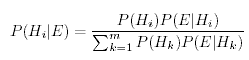Search

Useful Lists

Partners

Online Manuals

Implement Bayesian inference using PHP, Part 1
By Paul Meagher - 2004-04-21 Page:  1 2 3 4 5 6 7 8 9 10 11

## Deriving Bayes Theorem

You are now in a position to discuss the canonical formula for Bayes inference. The derivation of Bayes formula follows naturally from the definition of conditional probability using the probability format:

P(A | B) = P(A & B) / P(B)

Using some algebra, this equation can be rewritten as:

P(A & B) = P(A | B) P(B)

The same right-hand value can also be computed using A as the conditioning variable:

P(A & B) = P(B | A) P(A)

Given this equivalence, you can write:

P(A | B) P(B) = P(B | A) P(A)

Simplifying, you arrive at Bayes theorem:

P(A | B) = P(B | A) P(A) / P(B)

Notice that this formula for computing a conditional probability is similiar to the original formula with the exception that the joint probability P(A & B) that used to appear in the numerator has been replaced with the equivalent expression P(B | A) P(A).

## Computing the full posterior

Bayesian inference is often put forth as a prescriptive framework for hypothesis testing. Using this framework, it is standard to replace P(A | B) with P(H | E) where H stands for hypothesis and E stands for evidence. Bayes inference rule then looks like this:

P(H | E) = P(E | H) P(H) / P(E)

In words, the formula says that the posterior probability of a hypothesis given the evidence P(H | E) is equal to the likelihood of the evidence given the hypothesis P(E | H) multiplied by the prior probability of the hypothesis P(H). You can ignore P(E) as only serving a normalization role (in other words, ensuring the sum of all the cell probabilities is 1.0). You can thus mentally simplify the equation to:

P(H | E) = P(E | H) P(H)

The prior distribution P(H) in this equation can be represented in PHP as an indexed array of probability values (as shown):

 ``var \$priors = array();``

The `\$priors` array is expected to contain a list of numbers denoting the prior probability of each hypothesis. In the context of medical diagnosis, the `\$priors` array might contain the prevalence rates of each hypothesized disease in the population. Alternatively, the array might contain a medical specialist's best guess as to the prior probability of each disease under consideration given everything they know about each disease and current conditions.

The exact nature of the full posterior probability computation is made clearer by seeing that the posterior and likelihood terms appear in a PHP implementation as two-dimensional arrays (the closest you can currently get to a matrix datatype in PHP).

Listing 3. The posterior and likelihood terms appear in a PHP implementation as 2D arrays
 ````````````

For now, ignore the issue of how the `\$prior`, `\$likelihood`, and `\$evidence` distribution values are computed from raw data. You can posit magical `get` functions to obtain these values. The previous code shows how the values of the posterior probability matrix are computed by looping over the evidence items and the hypothesis alternatives.

The order of the index elements \$e and \$h in the posterior matrix might be puzzling until you realize that in PHP the evidence key should appear first in the posterior matrix because it is a lookup key. If you access the posterior matrix using an evidence key \$e, it will return an array containing the probability of each hypothesis under consideration (such as, +cancer, -cancer) given the particular evidence key you have supplied (like +test). The code above computes the full posterior distribution over all evidence keys. To compute a row of the full posterior distribution for a particular evidence key, you would use this formula:

Figure 2. Formula to compute posterior distributionView Implement Bayesian inference using PHP, Part 1 Discussion

Page:  1 2 3 4 5 6 7 8 9 10 11 Next Page: Medical diagnosis wizard